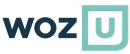Home>Courses>Basic Statistics## Basic Statisticsbeginner

The Basic Statistics course will help students gain a fundamental understanding of statistical concepts used throughout the Data Science program. Topics covered include probability, data types, common distributions, common descriptive statistics, and statistical inference. Objectives: Use probability rules and Bayes Theorem. Explain the difference between continuous and categorical variables. Use common descriptive statistics for both populations and samples. Use common distributions including Uniform, Normal, z-distribution, t-distribution, F-distribution, Poisson, and Weibul. Use the Central Limit Theorem. Explain what statistical inference is, and how to create confidence intervals. Demonstrate high-level data exploration.

### Blog